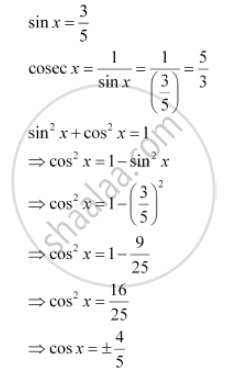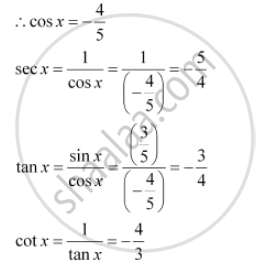Share

# Find the Values of Other Five Trigonometric Functions If Sin X = 3/5 X Lies In Quadrant - Mathematics

#### Question

Find the values of other five trigonometric functions if sin x = 3/5 x lies in quadrant

#### SolutionSince x lies in the 2nd quadrant, the value of cos x will be negativeIs there an error in this question or solution?
Find the Values of Other Five Trigonometric Functions If Sin X = 3/5 X Lies In Quadrant Concept: Signs of Trigonometric Functions.